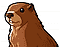# mORMot Open Source

You are not logged in.

## #1 2020-06-01 13:22:18

ASiwon
Member
From: Poland
Registered: 2015-01-30
Posts: 82

### Problem with saving date values to the Oracle in batch mode.

Hi,

I'm using mORMot with Oracle database connected via SynDbOracle. Few days ago I decided to change my process to use batch mode to improve the performance.

After changing the code to use batch mode the program has started to reports databases constraints rules violation for columns with date values. But they occurs only when the Oracle database is used. For SQLite database everything works perfectly.

After long investigation I found the reason. Here is a little long explanation.
In SynDBOracle unit to the execution of queries the procedure ExecutePrepared is used. Here is the piece of code which is used to set date values to the query params:

``````          for j := 0 to fParamsArrayCount-1 do
if VArray[j]='null' then // bind null (ftUTF8 should be '"null"')
aIndicator[i][j] := -1 else begin
if VDBType=SQLT_INT then
SetInt64(pointer(Varray[j]),oDataINT^[j]) else
case VType of
ftUTF8,ftDate: begin
L := length(VArray[j])-2; // -2 since quotes will be removed
if VType=ftDate then
if L<=0 then
oDataDAT^[j].From(PUTF8Char(pointer(VArray[j]))+1,L) else      <--- Here is the date conversion command
if L>oLength then
if L*fParamsArrayCount>MAX_INLINED_PARAM_SIZE then
raise ESQLDBOracle.CreateUTF8(
'%.ExecutePrepared: Array parameter #% STR too big',[self,i+1]) else
oLength := L;
end;``````

this code runs procedure TOracleDate.From to read date value from the JSON string to the TOracleDate record. In procedure From is used function: Iso8601ToTimeLogPUTF8Char which not always works correctly in this place.

``````procedure TOracleDate.From(aIso8601: PUTF8Char; Length: integer);
var Value: QWord;
Value32: cardinal absolute Value;
Y: cardinal;
NoTime: boolean;
begin
PInteger(PtrUInt(@self)+3)^ := 0; // set Day=Hour=Min=Sec to 0
Value := Iso8601ToTimeLogPUTF8Char(aIso8601,Length,@NoTime);
if Value=0 then begin
PInteger(@self)^ := 0;
exit; // invalid ISO-8601 text -> store as null date
end;
...``````

It is because the Iso8601ToTimeLogPUTF8Char function expects date value in format: 2000-01-01 but in this case to the function is passed string from JSON format and it has single quote char
on the end of the string. The string with date looks like: 2000-01-01'. The function expects that after date part will be started time part or the end of the string.
When the function finds single quote char it stops the execution and returns 0. This causes the function for any date string returns value 0 and all these wrong values are saved to the database.

For my purposes I have change the Iso8601ToTimeLogPUTF8Char function code:

``````function Iso8601ToTimeLogPUTF8Char(P: PUTF8Char; L: integer; ContainsNoTime: PBoolean): TTimeLog;
// bits: S=0..5 M=6..11 H=12..16 D=17..21 M=22..25 Y=26..40
// i.e. S<64 M<64 H<32 D<32 M<16 Y<9999: power of 2 -> use fast shl/shr
var V,B: PtrUInt;
tab: {\$ifdef CPUX86NOTPIC}TNormTableByte absolute ConvertHexToBin{\$else}PNormTableByte{\$endif};
begin
result := 0;
if P=nil then
exit;
if L=0 then
L := StrLen(P);
if L<4 then
exit; // we need 'YYYY' at least
if P='T' then
dec(P,8) else begin // 'YYYY' -> year decode
{\$ifndef CPUX86NOTPIC}tab := @ConvertHexToBin;{\$endif} // faster on PIC/x86_64
V := tab[ord(P)];
if V>9 then exit;
B := tab[ord(P)];
if B>9 then exit else V := V*10+B;
B := tab[ord(P)];
if B>9 then exit else V := V*10+B;
B := tab[ord(P)];
if B>9 then exit else V := V*10+B;
result := Int64(V) shl 26;  // store YYYY
if P in ['-','/'] then begin inc(P); dec(L); end; // allow YYYY-MM-DD
if L>=6 then begin // YYYYMM
V := ord(P)*10+ord(P)-(48+480+1); // Month 1..12 -> 0..11
if V<=11 then
inc(result,V shl 22) else begin
result := 0;
exit;
end;
if P in ['-','/'] then begin inc(P); dec(L); end; // allow YYYY-MM-DD
if L>=8 then begin  // YYYYMMDD
V := ord(P)*10+ord(P)-(48+480+1); // Day 1..31 -> 0..30
if (V<=30) and(P in [#0,' ','T', '''']) then  <-- here is single quote exception added.``````

after this change date values are correctly saved to the database. I could add this correction to the pull request, but I'm not sure is it the best place to make correction for this problem.

best regards

Offline

## #2 2020-06-01 13:46:41

abFrom: France
Registered: 2010-06-21
Posts: 14,039
Website

### Re: Problem with saving date values to the Oracle in batch mode.

I guess https://synopse.info/fossil/info/b28bbab8c9 is more correct.
Thanks for the feedback.

But please follow the forum rules, and don't put such an amount of code within the thread itself.

Offline